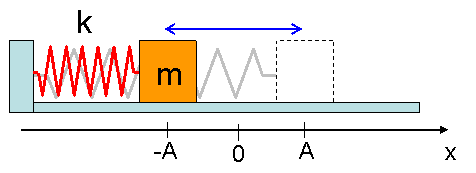# Problem: A mass on a spring moves with simple harmonic motion as shown.1)Where is the acceleration of the mass most positive?x = -Ax = 0x = +A

###### FREE Expert Solution

Acceleration of mass in a simple harmonic motion is given by:

$\overline{){\mathbf{a}}{\mathbf{=}}{\mathbf{-}}{{\mathbf{\omega }}}^{{\mathbf{2}}}{\mathbf{x}}}$

88% (433 ratings)###### Problem Details

A mass on a spring moves with simple harmonic motion as shown.1)Where is the acceleration of the mass most positive?
x = -A
x = 0
x = +A